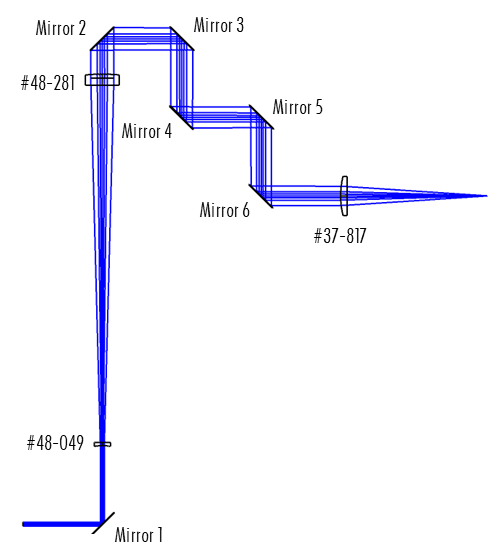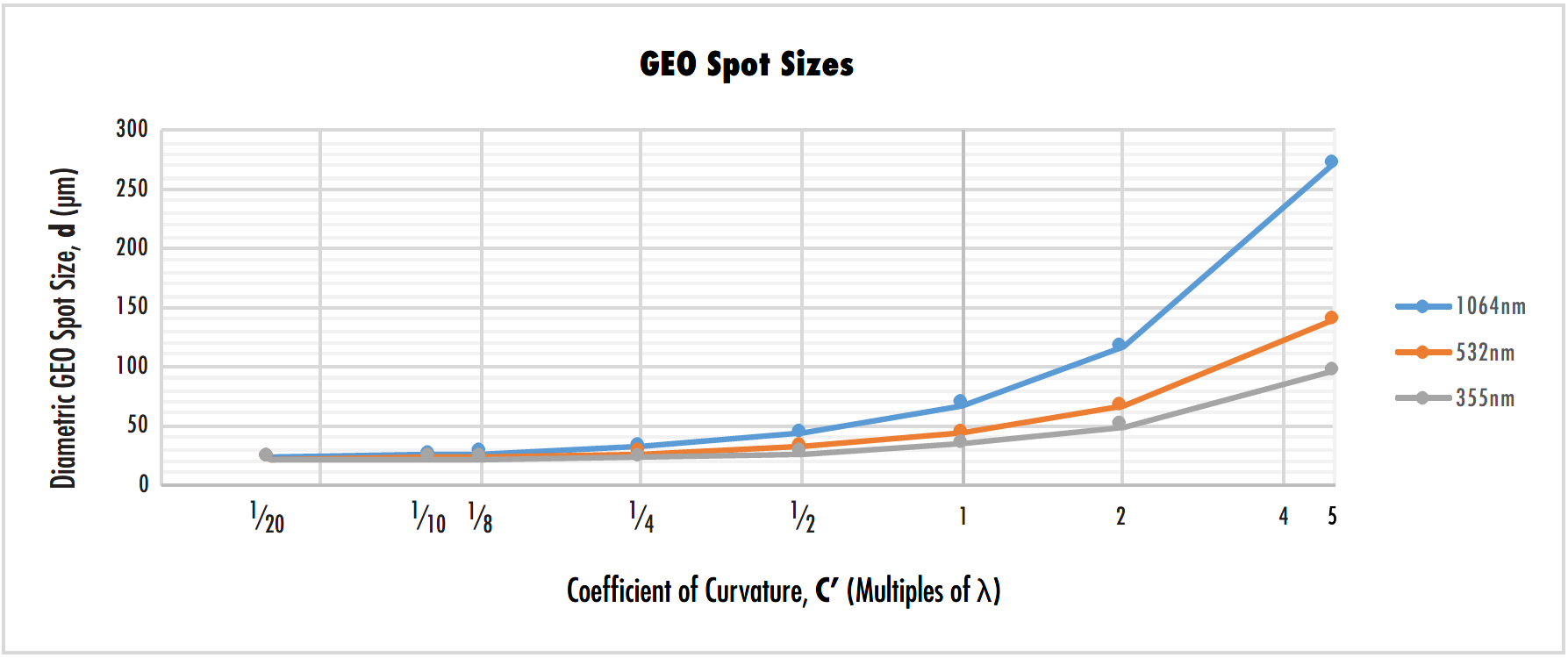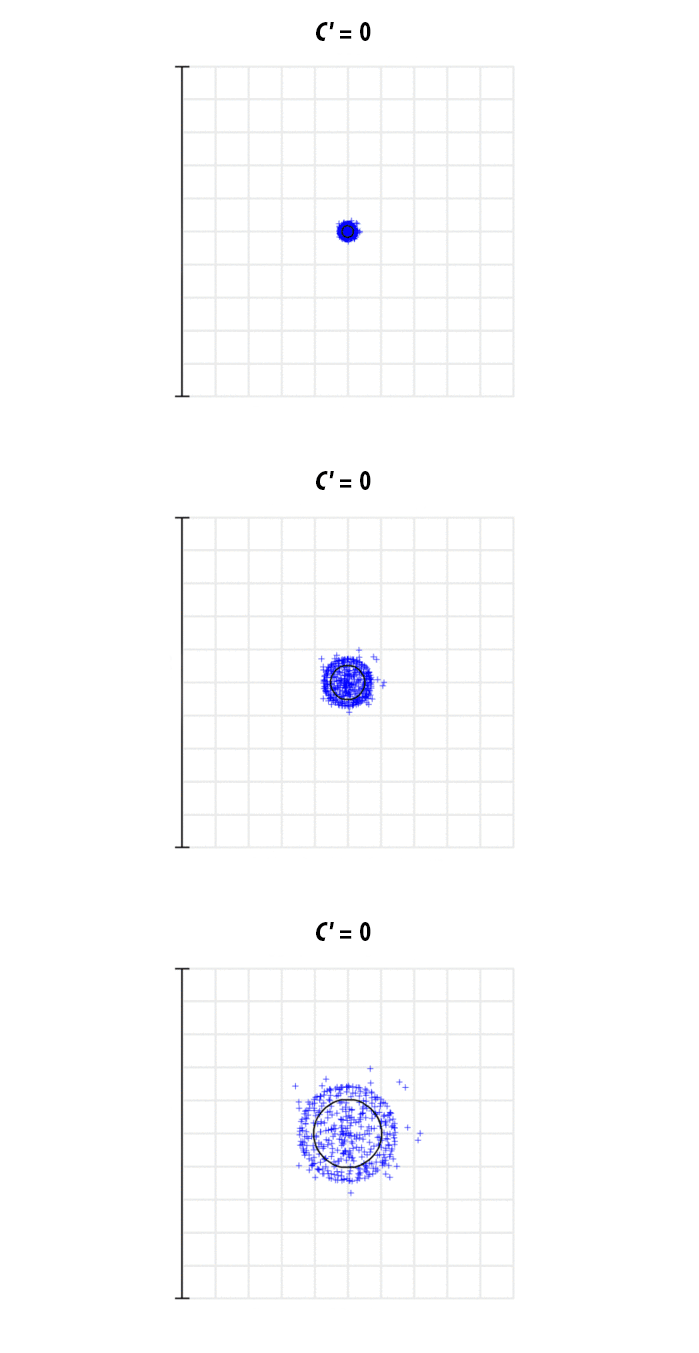# Effects of Laser Mirror Surface Flatness

Laser systems often require extremely precise optical components, such as mirrors and lenses, with specifications that approach the limits of what is possible to manufacture. When creating an optical system in which a laser will be used, it is important to manage one’s expectations about what is manufacturable at the price point desired. Understanding which performance factors are crucial versus which factors are desired, but unnecessary, can save time and money. Depending on the application, a laser system may or may not require high-performance custom optical components. This application note will demonstrate this point by exploring the simulated effects of mirror surface flatness on the focused spot size of an input Gaussian laser beam propagating though a simulated laser materials processing system.

This application note has implications for any laser system including one or more laser mirrors. To maximize laser system efficiency, laser mirrors are usually coated with ion-beam-sputtered (IBS) coatings or evaporative coatings. IBS coatings are popular mirror coating types for their extremely high spectral performance, meaning that they can achieve very high reflectivities. However, they do put very high stress on the surface to which they are applied, potentially warping the surface of the mirror unless a compensating coating on the backside of the optic or a lengthy annealing is applied. Evaporative coatings only apply a small amount of stress to the mirror surface, but their spectral performance is lower than that of IBS coatings. While this application note does not directly explore the effects of coatings, the simulated mirror curvatures approximate curvatures imparted to mirrors from the symmetrical stresses of an IBS coating.

## Optical Limits and Guidelines

Surface flatness and curvature both refer to the shape of the surface of a mirror. While surface flatness is the deviation of the surface from its nominal form, curvature is defined as the reciprocal of the radius of curvature (Equation 1).

(1)$$C = \frac{1}{R}$$

Because this simulation requires the radius of curvature, $\small{R}$, to be input, it must be determined from several other dimensions and properties of the mirror. It is first assumed that the mirror will have a spherical curvature, which is often the case for stressed coatings, and that the maximum sag, the abbreviation for sagitta, will be exactly equal to the surface flatness, $\small{\text{SF}}$ (Equation 2).

(2)\begin{align} \text{sag} & = \text{SF} \left[ \text{waves} \right] \\ & = \text{SF} \left[ \lambda \right] \end{align}

In the case where a beam reflects off a single mirror, the surface flatness will also be equal to the reflected wavefront error, or wavefront distortion. Surface flatness typically is reported in units of fringes or waves and, as such, the surface flatness of a mirror is reported as a positive real fraction or whole number multiple of the wavelength of light being used with the mirror. Expanding the equation for sag requires precise attention to semantics. Equation 3 requires the mirror clear aperture semi-diameter, $\small{\rho}$, which is also known as the clear semi-diameter.

(3)$$R - \sqrt{R^2 - \rho^2} = \text{SF}$$

This distinction is important to make for the sake of an accurate simulation in Zemax OpticStudio as there also exists a mechanical semi-diameter, which is simply half the optic diameter and does not factor in the clear aperture. With the surface flatness and the clear semi-diameter, the radius of curvature can be determined (Equation 4).

(4)$$R = \frac{1}{2} \left[ \text{SF} + \frac{\rho ^2}{\text{SF}} \right]$$

Since the sag of the mirror is many magnitudes smaller than the radius of the mirror, the contribution of the first term to the radius of curvature is insignificant $\left( \frac{\rho ^2}{\text{SF}} >> \text{SF} \right)$. It is therefore reasonable to use the parabolic approximation to simplify Equation 4 to Equation 5.

(5)$$R \approx \frac{1}{2} \times \frac{\rho ^2}{\text{SF}}$$

Because curvature is inversely proportional to the radius of curvature, the curvature is directly proportional to the surface flatness. This is important to distinguish because mirror curvature will be changed by only changing the surface flatness in this application note. For example, a mirror with a diameter of 25mm has a mechanical semi-diameter of 12.5mm. With a clear aperture of 85% the clear semi-diameter $\small{\left( \rho \right)}$ of the mirror is 10.625mm. With a surface flatness of $\tfrac{\lambda}{10}$ (also stylized as $\small{\lambda}$/10), and using a wavelength of 1064nm, the radius of curvature is approximately 5.3 × 105mm and the curvature is 1.9 × 10-6mm.

### The Diffraction Limit

The diffraction limit of the focused spot, described by the Airy disk equation, can be used to determine the lower limit for which the diameter spot size of a beam can be focused, denoted as d and given the wavelength of the light, $\small{\lambda}$, the focal length of the converging lens, $\small{f}$, and the size or diameter of the beam, $\small{D}$. This lower limit is determined by assuming the converging lens is a perfect, paraxial lens free of any aberration (Equation 6).

(6)$$d \approx 2.44 \frac{f \, \lambda }{D}$$

For example, a 1064nm laser beam with a diameter of 5mm being focused by a 100mm focal length paraxial lens, has an Airy spot diameter of approximately 52μm. A beam of light cannot be focused any smaller without the use of a lens with greater power, however, because perfect aberration-free paraxial lenses do not truly exist, the spot size will likely be greater than this value. The Airy disk equation simply provides the lower limit, known as the diffraction limit, to which a spot size can be focused.

### Zemax OpticStudio Simulation & Results

The simulation used to test the effects of mirror curvature on the focused beam size was created in Zemax OpticStudio 18.9. The model uses a 2.5mm diameter Gaussian beam of a given wavelength passing through a system consisting of six identical mirrors (having the same diameters and surface flatnesses) and three Edmund Optics lenses, as depicted in Figure 1.##### Figure 1: This simulated beam expander system features six identical mirrors of variable curvature, PCV lens #48-049 and PCX lens #48-281 as the 5X beam expander, and PCX lens #37-817 as the condenser lens

In this simulation, the mirrors were given mechanical semi-diameters of 12.5mm and clear semi-diameters of 10.625mm to adhere to the convention, which advises that the clear aperture for an optic should be 85% of its diameter. This diameter was selected to ensure that the ray bundle would be within the clear aperture of the second lens in the laser beam expander, though in a real-world application much larger diameters would be chosen for this beam size to avoid clipping of the Gaussian beam. The 5X laser beam expander consisted of Edmund Optics lenses #48-049 and #48-281. Mirrors 2 through 6 were placed 50mm away from each other to fold the optical path, simulating space constraints in a real laser system. The distance between the two lenses consisting of the laser beam expander, as well as the distance from the last mirror to the condenser lens, were optimized to focus the beam at the final focus plane to obtain the geometric (GEO) spot sizes for the focused beam at varied mirror curvatures.

In order to evaluate the spot size at different surface flatness values, but the same wavelength, the surface flatness was defined as a positive real number multiple of the wavelength, and this multiple was increased. This multiple will be called the coefficient of curvature, $\small{C'}$, for simplicity. This coefficient of curvature, which is a unitless value, is not to be confused with curvature, which is the reciprocal of the radius of curvature and has units of length, as given in Equation 1. Curvature also can span multiple magnitudes, whereas the coefficient of curvature, which can be any positive number, is usually a fraction less than 1. Thus, the sag can be represented as the wavelength of light in the system, $\small{\lambda}$, multiplied by the coefficient of curvature (Equation 7).

(7)\begin{align} C' & \in \mathbb{R}_{\geq 0} \\ \text{SF} \left[ \lambda \right] & = C' \\ \text{SF} \left[ \text{nm} \right] & = C' \times \lambda \end{align}

The respective results for three wavelengths and nine coefficients of curvature values are shown in Table 1 and Figure 2.

 GEO Spot Sizes [μm] Surface Flatness (P-V) $0 \lambda$ $\frac{\lambda}{20}$ $\frac{\lambda}{10}$ $\frac{\lambda}{8}$ $\frac{\lambda}{4}$ $\frac{\lambda}{2}$ $1 \lambda$ $2 \lambda$ $5 \lambda$ 1064nm 22.4 22.9 25.1 26.3 32.0 43.7 67.2 116.4 270.8 532nm 21.7 21.6 22.3 22.8 25.6 31.3 42.9 66.1 139.0 355nm 20.7 21.6 21.6 21.9 22.7 26.5 34.1 34.1 96.1
##### Table 1: Simulated spot sizes for increasing mirror curvature at 1064nm and the first two Nd:YAG harmonic wavelengths##### Figure 2: Geometric spot sizes from Table 1 quickly grow as mirrors become more curved

Figure 2 shows that the focused spot sizes for mirrors with a $\tfrac{\lambda}{20}$ surface flatness on a 300μm scale appear to be almost exactly the same. As surface flatness worsens, focused spot sizes become larger as for longer wavelengths. After a surface flatness of $\tfrac{\lambda}{4}$, the focused spot size starts to increase more noticeably for all wavelengths.

### Key Effects and Recommendations

In reality, there are no perfectly flat mirrors. With looser surface flatness specifications (increased mirror curvature), spot size increases and the reflected wavefront degrades. When the surface profile of a mirror varies significantly from its nominal form, it can be helpful to fit the aberrations on the surface to a set of functions called Zernike polynomials. Depending on the exact surface profile of the mirror and the angles at which the mirrors are positioned with respect to the incident beam, aberration effects from downstream lenses and mirrors can aggregate constructively or destructively.

In this simulation, for simplicity, mirrors were treated as only spherical or flat. As a result, beam profiles became less circular and more spread out. It is, however, important to note that spherical aberrations were originally present in the laser beam expander and beam focusing lenses and the effects inevitably were carried through the system and distorted by the tilt and spherical curvature of the mirrors. Because these interactions are complex and could be potentially additive or subtractive depending on where the mirrors are placed, OpticStudio optimization of lens spacing was needed to minimize aberration effects and focus the spot. Another factor not considered was the use of optical coatings.

Also important to not overlook is how the wavelength of light in conjunction with the mirror curvature affects the spot size and shape. At larger wavelengths, deviations in spot size and shape are larger and appear more pronounced than at shorter wavelengths (Figure 3).##### Figure 3: The focused spot size of a 1064nm beam at increasing coefficients of curvature at three scales: 600μm (top), 200μm (middle), and 100μm (bottom)

Because this simulation was strictly theoretical, each mirror and lens reflected and transmitted 100% of the incident light, respectively. In reality, laser systems use high-performance reflective coatings to maximize mirror reflectance and anti-reflection coatings to maximize lens transmission. These coatings, however, have specified wavelengths or wavelength ranges and laser-induced damage thresholds (LIDTs) that must be considered for real systems.

It should be noted that a large number of specifications and factors can drive system performance and manufacturing costs, such as coating types or mechanical specifications such as parallelism or surface accuracy. These additional factors are just as important to consider when selecting components for a laser system, though many will be omitted from the bulk of this simulation.

### Looking to learn about optics and optical design?

Learn the basics of optics needed to begin designing optical systems in this educational, online Fundamentals of Optics course co-created by Edmund Optics and Zemax. It does not require any previous knowledge in the field of optics.

REGISTER NOW